# Optimization

Highly-configurable non-linear optimization framework for quantum control

The Q-CTRL Python package exposes a highly-flexible optimization engine for general-purpose gradient-based optimization.

The optimization engine provides a large, modular collection of configuration options in order to support a wide variety of systems and constraints relevant to quantum control. These options can be combined in different ways to produce optimization solutions tailored to specific systems. In this guide we demonstrate optimization using several different types of systems and constraints, to give a taste of the available flexibility. All the techniques presented here can be cross-combined to yield an even wider class of supported configurations.

## Imports and initialization

# Essential imports
import numpy as np
from qctrl import Qctrl

# Plotting imports
from qctrlvisualizer import plot_controls
import matplotlib.pyplot as plt

# Starting a session with the API
qctrl = Qctrl()


## Worked example: robust control of a single qubit

We first present a detailed worked example of robust optimization in a single-qubit system. Specifically, we consider a single-qubit system represented by the following Hamiltonian:

\begin{align*} H(t) &= \frac{\nu}{2} \sigma_{z} + \frac{1}{2}\left(\gamma(t)\sigma_{-} + \gamma^*(t)\sigma_{+}\right) + \frac{\alpha(t)}{2} \sigma_{z} + \beta(t) \sigma_{z} \,, \end{align*}

where $\nu$ is the qubit detuning, $\gamma(t)$ and $\alpha(t)$ are, respectively, complex and real time-dependent pulses, and $\beta(t)$ is a small, slowly-varying stochastic dephasing noise process and $\sigma_{\pm}$ are the qubit ladder operators and $\sigma_{z}$ is the Pauli-Z operator.

The functions of time $\gamma(t)$ and $\alpha(t)$ are not predetermined, and instead are optimized by the Q-CTRL optimization engine in order to achieve some target operation.

### Defining the graph

The flexible Q-CTRL optimization engine expresses all optimization problems as data flow graphs, which describe how optimization variables (variables that can be tuned by the optimizer) are transformed into the cost function (the objective that the optimizer attempts to minimize).

Below we show how to create a data flow graph for optimizing the single-qubit system described above. Comments in the code explain the details of each step.

# Define standard matrices
sigma_y = np.array([[0, -1j], [1j, 0]])
sigma_z = np.array([[1, 0], [0, -1]])
sigma_m = np.array([[0, 1], [0, 0]])

# Define physical constants
nu = 2*np.pi * 0.5 * 1e6 #Hz
gamma_max = 2*np.pi * 0.5e6 #Hz
alpha_max = 2*np.pi * 0.5e6 #Hz
segment_count = 50
duration = 10e-6 #s

# Define the data flow graph describing the system
with qctrl.create_graph() as graph:

# Create a constant piecewise-constant (PWC) operator representing the
# detuning term
detuning = qctrl.operations.constant_pwc_operator(
duration=duration,
operator=nu * sigma_z / 2,
)

# Create a complex PWC signal, with optimizable modulus and phase,
# representing gamma(t)
gamma = qctrl.operations.complex_pwc_signal(
moduli=qctrl.operations.bounded_optimization_variable(
count=segment_count, lower_bound=0, upper_bound=gamma_max,
),
phases=qctrl.operations.unbounded_optimization_variable(
count=segment_count,
initial_lower_bound=0,
initial_upper_bound=2*np.pi,
),
duration=duration,
name="gamma",
)
# Create a PWC operator representing the drive term
drive = qctrl.operations.pwc_operator_hermitian_part(
qctrl.operations.pwc_operator(signal=gamma, operator=sigma_m)
)

# Create a real PWC signal, with optimizable amplitude, representing
# alpha(t)
alpha = qctrl.operations.pwc_signal(
values=qctrl.operations.bounded_optimization_variable(
count=segment_count, lower_bound=-alpha_max, upper_bound=alpha_max
),
duration=duration,
name="alpha",
)
# Create a PWC operator representing the clock shift term
shift = qctrl.operations.pwc_operator(signal=alpha, operator=sigma_z / 2)

# Create a constant PWC operator representing the dephasing noise
# (note that we scale by 1/duration to ensure consistent units between
# the noise Hamiltonian and the control Hamiltonian)
dephasing = qctrl.operations.constant_pwc_operator(
duration=duration,
operator=sigma_z / duration)

# Create the target operator
target_operator = qctrl.operations.target(operator=sigma_y)

# Create infidelity
infidelity = qctrl.operations.infidelity_pwc(
hamiltonian=qctrl.operations.pwc_sum([detuning, drive, shift]),
noise_operators=[dephasing],
target_operator=target_operator,
name="infidelity",
)


### Running an optimization

With the graph object created, an optimization can be run using the qctrl.functions.calculate_optimization function. The cost, the outputs, and the graph must be provided. The function returns the results of the optimization.

optimization_result = qctrl.functions.calculate_optimization(
cost_node_name="infidelity",
output_node_names=["alpha", "gamma"],
graph=graph,
)

100%|██████████| 100/100 [00:11<00:00,  8.67it/s]

print("Optimized cost:\t", optimization_result.cost)

Optimized cost:	 5.210844473342732e-14


### Visualizing the pulses

We may use the qctrlvisualizer package to plot the optimized pulses, which are available in the result object.

plot_controls(plt.figure(),
controls={"$\\alpha$": optimization_result.output["alpha"],
"$\\gamma$": optimization_result.output["gamma"]})
plt.show()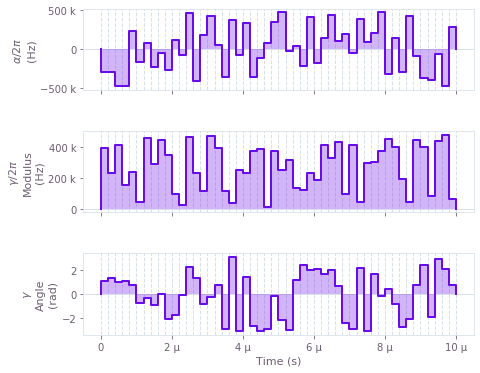## Example: time-symmetrized pulses

Next we show how time symmetry can be incorporated into the optimization problem. In some cases, forcing control pulses to exhibit time symmetry can yield natural noise robustness while simplifying the optimization problem (by halving the dimensionality of the search space). In this example, we perform a robust optimization of a single qubit using symmetric pulses. The single-qubit system is represented by the following Hamiltonian:

\begin{align*} H(t) &= \frac{\nu}{2} \sigma_{z} + \frac{1}{2}\left(\gamma(t)\sigma_{-} + \gamma^*(t)\sigma_{+}\right) + \frac{\alpha(t)}{2} \sigma_{z} + \beta(t) \sigma_{z} \,, \end{align*}

where $\nu$ is the qubit detuning, $\gamma(t)$ and $\alpha(t)$ are, respectively, complex and real time-dependent pulses, and $\beta(t)$ is a small, slowly-varying stochastic dephasing noise process.

To enforce time symmetry, we create the control signals in two steps: we first create a standard signal for the first half of the gate duration, and then we create a copy (depending on the same underlying control parameters) that gets reflected and concatenated with the original signal.

# Define standard matrices
sigma_y = np.array([[0, -1j], [1j, 0]])
sigma_z = np.array([[1, 0], [0, -1]])
sigma_m = np.array([[0, 1], [0, 0]])

# Define physical constraints
gamma_max = 2 * np.pi * 8.5e6 #Hz
alpha_max = 2 * np.pi * 8.5e6 #Hz
nu = 2 * np.pi * 6e6 #Hz
segment_count = 50
duration = 154e-9 #s

# Create graph object
with qctrl.create_graph() as graph:

# Create detuning term
detuning = qctrl.operations.constant_pwc_operator(
duration=duration,
operator=nu * sigma_z / 2,
)

# Create a complex PWC signal describing the first half of gamma(t)
half_gamma = qctrl.operations.complex_pwc_signal(
moduli=qctrl.operations.bounded_optimization_variable(
count=segment_count, lower_bound=0, upper_bound=gamma_max
),
phases=qctrl.operations.unbounded_optimization_variable(
count=segment_count,
initial_lower_bound=0,
initial_upper_bound=2 * np.pi,
),
duration=duration / 2,
)
# Define gamma(t) by symmetrizing half_gamma
gamma = qctrl.operations.symmetrize_pwc(
half_gamma,
name="gamma",
)
# Create drive term
drive = qctrl.operations.pwc_operator_hermitian_part(
qctrl.operations.pwc_operator(signal=gamma,
operator=sigma_m)
)

# Create alpha(t) similarly
alpha = qctrl.operations.symmetrize_pwc(
qctrl.operations.pwc_signal(
values=qctrl.operations.bounded_optimization_variable(
count=segment_count, lower_bound=-alpha_max, upper_bound=alpha_max
),
duration=duration / 2,
),
name="alpha",
)
# Create clock shift term
shift = qctrl.operations.pwc_operator(signal=alpha,
operator=sigma_z / 2)

# Create dephasing noise term
dephasing = qctrl.operations.constant_pwc_operator(
duration=duration,
operator=sigma_z / duration)

# Create target
target_operator = qctrl.operations.target(operator=sigma_y)

# Create infidelity
infidelity = qctrl.operations.infidelity_pwc(
qctrl.operations.pwc_sum([detuning, drive, shift]),
target_operator,
[dephasing],
name="infidelity"
)

# Run the optimization
optimization_result = qctrl.functions.calculate_optimization(
cost_node_name="infidelity",
output_node_names=["alpha", "gamma"],
graph=graph,
)

print("Optimized cost:\t", optimization_result.cost)

# Plot the optimized controls
plot_controls(plt.figure(),
controls={"$\\alpha$": optimization_result.output["alpha"],
"$\\gamma$": optimization_result.output["gamma"]})
plt.show()

100%|██████████| 100/100 [00:19<00:00,  5.21it/s]

Optimized cost:	 6.737233903202292e-12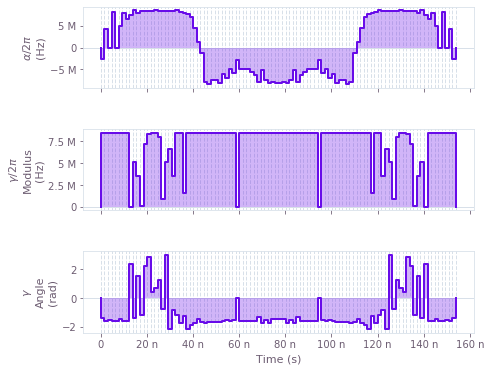## Example: non-linear dependence on control pulses

We next present an example showing how a system with non-linear dependence between controls and Hamiltonian may be optimized. We consider an artificial single-qubit system represented by the following Hamiltonian:

\begin{align*} H(t) &= \alpha_1(t)\sigma_x + \frac{\alpha_1(t)^2}{\alpha_{\text{max}}}\sigma_y + \alpha_2(t)\sigma_z \,, \end{align*}

where $\alpha_1(t)$ and $\alpha_2(t)$ are real time-dependent pulses, and we note the non-linear dependence of the Hamiltonian on $\alpha_1(t)$. While here we have chosen a simple artificial non-linearity for the sake of convenience and brevity, the approach we demonstrate extends trivially to far more complex, physically-relevant situations.

Expressing non-linear dependence between control parameters and Hamiltonians is achieved by performing standard arithmetical operations on the control parameters prior to constructing signals. In this case the operations are very simple—we simply square and re-scale the parameters describing the $\alpha_1(t)$ values—but in general any combination of supported primitive operations may be used.

# Define Pauli matrices
sigma_x = np.array([[0, 1], [1, 0]])
sigma_y = np.array([[0, -1j], [1j, 0]])
sigma_z = np.array([[1, 0], [0, -1]])

# Define physical constraints
alpha_max = 2*np.pi * 6e6 #Hz
segment_count = 40
duration = 200e-9 #s

# Create graph object
with qctrl.create_graph() as graph:

# Create the values backing the alpha_1(t) signal
alpha_1_values = qctrl.operations.bounded_optimization_variable(
count=segment_count,
lower_bound=-alpha_max,
upper_bound=alpha_max,
)

# Create the alpha_1(t) signal
alpha_1 = qctrl.operations.pwc_signal(
values=alpha_1_values, duration=duration, name="alpha_1"
)

# Apply a non-linear transformation to create the alpha_1_squared(t) signal
alpha_1_squared_values = alpha_1_values * (alpha_1_values / alpha_max)
alpha_1_squared = qctrl.operations.pwc_signal(
values=alpha_1_squared_values, duration=duration, name="alpha_1_squared"
)

# Create the alpha_2(t) signal
alpha_2 = qctrl.operations.pwc_signal(
values=qctrl.operations.bounded_optimization_variable(
count=segment_count, lower_bound=-alpha_max, upper_bound=alpha_max
),
duration=duration,
name="alpha_2",
)

# Create Hamiltonian terms
x_term = qctrl.operations.pwc_operator(signal=alpha_1, operator=sigma_x)
y_term = qctrl.operations.pwc_operator(signal=alpha_1_squared, operator=sigma_y)
z_term = qctrl.operations.pwc_operator(signal=alpha_2, operator=sigma_z)

target_operator = qctrl.operations.target(operator=sigma_x)

# Create infidelity
infidelity = qctrl.operations.infidelity_pwc(
qctrl.operations.pwc_sum(terms=[x_term, y_term, z_term]),
target_operator=target_operator,
name="infidelity",
)

# Run the optimization
optimization_result = qctrl.functions.calculate_optimization(
cost_node_name="infidelity",
output_node_names=["alpha_1", "alpha_1_squared", "alpha_2"],
graph=graph,
)

print("Optimized cost:\t", optimization_result.cost)

# Plot the optimized controls
plot_controls(plt.figure(),
controls={"$\\alpha_1$": optimization_result.output["alpha_1"],
"$\\alpha_1^2$": optimization_result.output["alpha_1_squared"],
"$\\alpha_2$": optimization_result.output["alpha_2"]})
plt.show()

100%|██████████| 100/100 [00:05<00:00, 17.35it/s]

Optimized cost:	 1.7763568394002505e-15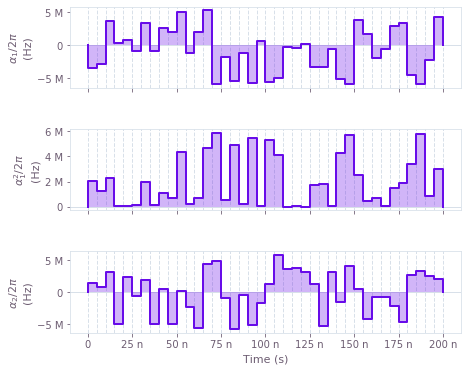## Example: band-limited pulses with bounded slew rates

Next we show how to optimize a system in which the rates of change of the controls are limited. Using this constraint can help to ensure that optimized controls can be reliably implemented on physical hardware (which may be subject to bandwidth limits). We consider a standard single-qubit system subject to dephasing noise:

\begin{align*} H(t) &= \frac{1}{2} \alpha_1(t)\sigma_{x} + \frac{1}{2} \alpha_2(t) \sigma_{z} + \beta(t) \sigma_{z} \,, \end{align*}

where $\alpha_1(t)$ and $\alpha_2(t)$ are real time-dependent pulse and $\beta(t)$ is a small, slowly-varying stochastic dephasing noise process. In this case, we enforce a maximum slew rate constraint on $\alpha_1(t)$ and $\alpha_2(t)$, to cap the variation between adjacent segment values.

To implement a bounded slew rate, we create signal values using a Q-CTRL helper function, qctrl.operations.anchored_difference_bounded_variables. This function produces values that are constrained to satisfy the slew rate requirement, and in addition are anchored to zero at the start and end of the gate.

# Define standard matrices
sigma_x = np.array([[0, 1], [1, 0]])
sigma_z = np.array([[1, 0], [0, -1]])
sigma_y = np.array([[0, -1j], [1j, 0]])

# Define physical constraints
alpha_max = 2 * np.pi * 8.5e6  # Hz
max_slew_rate = alpha_max / 10
segment_count = 250
duration = 400e-9  # s

# Create graph object
with qctrl.create_graph() as graph:

# Create alpha_1(t) signal
alpha_1_values = qctrl.operations.anchored_difference_bounded_variables(
count=segment_count,
lower_bound=-alpha_max,
upper_bound=alpha_max,
difference_bound=max_slew_rate,
)
alpha_1 = qctrl.operations.pwc_signal(
values=alpha_1_values, duration=duration, name="alpha_1"
)

# Create alpha_2(t) signal
alpha_2_values = qctrl.operations.anchored_difference_bounded_variables(
count=segment_count,
lower_bound=-alpha_max,
upper_bound=alpha_max,
difference_bound=max_slew_rate,
)
alpha_2 = qctrl.operations.pwc_signal(
values=alpha_2_values, duration=duration, name="alpha_2"
)

# Create drive term
drive = qctrl.operations.pwc_operator_hermitian_part(
qctrl.operations.pwc_operator(signal=alpha_1,
operator=sigma_x / 2)
)

# Create clock shift term
shift = qctrl.operations.pwc_operator(signal=alpha_2,
operator=sigma_z / 2)

# Create dephasing noise term
dephasing = qctrl.operations.constant_pwc_operator(
duration=duration,
operator=sigma_z / duration)

# Create target
target_operator = qctrl.operations.target(operator=sigma_y)

# Create infidelity
infidelity = qctrl.operations.infidelity_pwc(
qctrl.operations.pwc_sum([drive, shift]),
target_operator,
[dephasing],
name="infidelity",
)

# Run the optimization
optimization_result = qctrl.functions.calculate_optimization(
cost_node_name="infidelity",
output_node_names=["alpha_1", "alpha_2"],
graph=graph,
)

print("Optimized cost:\t", optimization_result.cost)

# Plot the optimized controls
plot_controls(plt.figure(),
controls={"$\\alpha_1$": optimization_result.output["alpha_1"],
"$\\alpha_2$": optimization_result.output["alpha_2"]})
plt.show()

100%|██████████| 100/100 [05:44<00:00,  3.45s/it]

Optimized cost:	 4.240427088198016e-13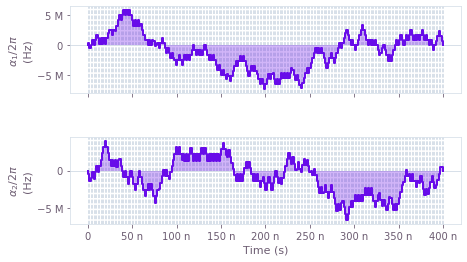## Example: band-limited pulses with filters

Next we show how to optimize a system using a different technique for enforcing pulse band limits, namely filtering. As above, producing band-limited controls can help to ensure that optimized controls can be reliably implemented on physical hardware, but in some cases one desires tighter control over the frequency domain behavior of the controls than what can be achieved with a slew rate bound. The technique we use here is to pass the controls through a linear time-invariant filter in order to enforce explicit bandwidth limits. We consider, once again, a standard single-qubit system subject to dephasing noise:

\begin{align*} H(t) &= \frac{1}{2} \alpha_1(t)\sigma_{x} + \frac{1}{2} \alpha_2(t) \sigma_{z} + \beta(t) \sigma_{z} \,, \end{align*}

where $\alpha_1(t)$ and $\alpha_2(t)$ are real time-dependent pulse and $\beta(t)$ is a small, slowly-varying stochastic dephasing noise process. In this case we force $\alpha_1(t)$ and $\alpha_2(t)$ to be signals produced from an ideal sinc filter, which forces the signals to exhibit a hard upper bound in the frequency domain.

To implement this behavior, we create optimizable signals as usual, and then use the qctrl.operations.convolve_pwc (to apply a filter) and qctrl.operations.discretize_stf (to obtain a piecewise-constant discretization of the smooth filtered signal) functions to derive band-limited signals. These band-limited signals are then used to construct the Hamiltonian.

# Define standard matrices
sigma_x = np.array([[0, 1], [1, 0]])
sigma_z = np.array([[1, 0], [0, -1]])
sigma_y = np.array([[0, -1j], [1j, 0]])

# Define physical constraints
alpha_max = 2 * np.pi * 8.5e6  #Hz
sinc_cutoff_frequency = 2 * np.pi * 48e6  #Hz
nu = 2 * np.pi * 6e6  #Hz
segment_count = 50
duration = 400e-9  #s

# Create graph object
with qctrl.create_graph() as graph:

# Create initial alpha_1(t) signal
alpha_1_values = qctrl.operations.bounded_optimization_variable(
count=segment_count,
lower_bound=-alpha_max,
upper_bound=alpha_max,
)
alpha_1 = qctrl.operations.pwc_signal(
values=alpha_1_values, duration=duration,
)
# Create filtered signal
alpha_1_filtered = qctrl.operations.convolve_pwc(
alpha_1,
qctrl.operations.sinc_integral_function(sinc_cutoff_frequency),
)
# Discretize to obtain smoothed alpha_1(t) signal
alpha_1 = qctrl.operations.discretize_stf(
stf=alpha_1_filtered,
duration=duration,
segments_count=segment_count,
name="alpha_1",
)

# Similarly, create alpha_2(t) signal
alpha_2_values = qctrl.operations.bounded_optimization_variable(
count=segment_count,
lower_bound=-alpha_max,
upper_bound=alpha_max,
)
alpha_2 = qctrl.operations.pwc_signal(
values=alpha_2_values, duration=duration,
)
# Create filtered signal
alpha_2_filtered = qctrl.operations.convolve_pwc(
alpha_2,
qctrl.operations.sinc_integral_function(sinc_cutoff_frequency),
)
# Discretize to obtain smoothed alpha_2(t) signal
alpha_2 = qctrl.operations.discretize_stf(
stf=alpha_2_filtered,
duration=duration,
segments_count=segment_count,
name="alpha_2",
)

# Create drive term
drive = qctrl.operations.pwc_operator_hermitian_part(
qctrl.operations.pwc_operator(signal=alpha_1,
operator=sigma_x / 2)
)

# Create clock shift term
shift = qctrl.operations.pwc_operator(signal=alpha_2,
operator=sigma_z / 2)

# Create dephasing noise term
dephasing = qctrl.operations.constant_pwc_operator(
duration=duration,
operator=sigma_z / duration)

# Create target
target_operator = qctrl.operations.target(operator=sigma_y)

# Create infidelity
infidelity = qctrl.operations.infidelity_pwc(
qctrl.operations.pwc_sum([drive, shift]),
target_operator,
[dephasing],
name="infidelity",
)

# Run the optimization
optimization_result = qctrl.functions.calculate_optimization(
cost_node_name="infidelity",
output_node_names=["alpha_1", "alpha_2"],
graph=graph,
)

print("Optimized cost:\t", optimization_result.cost)

# Plot the optimized controls
plot_controls(plt.figure(),
controls={"$\\alpha_1$": optimization_result.output["alpha_1"],
"$\\alpha_2$": optimization_result.output["alpha_2"]})
plt.show()

100%|██████████| 100/100 [00:12<00:00,  7.97it/s]

Optimized cost:	 4.7665771154585524e-12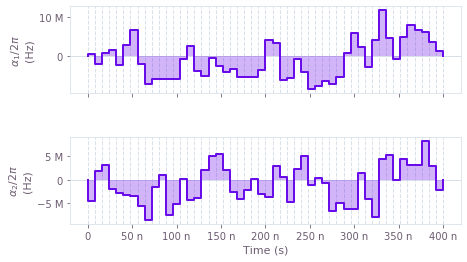## Example: incorporating smoothing via linear filters on control signals

We next present an example showing how systems containing linear time-invariant filters can be optimized. Unlike the example above, where we employed such filters in order to produce smooth controls, here we consider the case where such filters are present in the system itself. In this particular example, we consider the common scenario in which electronic filters are present on the control lines. Specifically, we consider a basic single-qubit system:

\begin{align*} H(t) &= \frac{1}{2} L(\alpha_1)(t)\sigma_{x} + \frac{1}{2} L(\alpha_2)(t) \sigma_{z} + \beta(t) \sigma_{z} \,, \end{align*}

where $\alpha_1(t)$ and $\alpha_2(t)$ are real time-dependent pulses, $\beta(t)$ is a small, slowly-varying stochastic dephasing noise process, and $L$ is a sinc filter applied to the pulses. The effect of these filters is to transform the control signals before they reach the quantum system, via convolution with the filter impulse response. Failing to take the filters into account during the optimization can lead to poor results, since in that case the system used for the optimization does not accurately model reality.

As above, we use the qctrl.operations.convolve_pwc function to apply a filter (obtained via the qctrl.operations.sinc_integral_function function) to a piecewise-constant signal. The resulting smooth tensor function (STF) may then be manipulated in exactly the same way as piecewise-constant functions, up to and including calculating the infidelity. We stress that even though highly irregular pulses are generated for $\alpha_1(t)$ and $\alpha_2(t)$, these pulses are chosen so that, when smoothed by the filters in the system, they will effect a high-quality gate.

# Define standard matrices
sigma_x = np.array([[0, 1], [1, 0]])
sigma_z = np.array([[1, 0], [0, -1]])

# Define physical constraints
alpha_max = 2*np.pi * 8.5e6 #Hz
nu = 2*np.pi * 6e6 #Hz
sinc_cutoff_frequency = 2*np.pi * 48e6 #Hz
segment_count = 50
duration = 250e-9 #s

# Create graph object
with qctrl.create_graph() as graph:
# Create alpha_1(t) signal
alpha_1_values = qctrl.operations.bounded_optimization_variable(
count=segment_count,
lower_bound=-alpha_max,
upper_bound=alpha_max,
)
alpha_1 = qctrl.operations.pwc_signal(
values=alpha_1_values, duration=duration, name="alpha_1",
)
# Create filtered signal
alpha_1_filtered = qctrl.operations.convolve_pwc(
alpha_1,
qctrl.operations.sinc_integral_function(sinc_cutoff_frequency),
)

# Similarly, create filtered alpha_2(t) signal
alpha_2_values = qctrl.operations.bounded_optimization_variable(
count=segment_count,
lower_bound=-alpha_max,
upper_bound=alpha_max,
)
alpha_2 = qctrl.operations.pwc_signal(
values=alpha_2_values, duration=duration, name="alpha_2",
)
alpha_2_filtered = qctrl.operations.convolve_pwc(
alpha_2,
qctrl.operations.sinc_integral_function(sinc_cutoff_frequency),
)

# Create drive term (note the use of STF functions instead of PWC functions,
# because we are dealing with smooth signals instead of piecewise-constant
# signals).
drive = qctrl.operations.stf_operator(alpha_1_filtered, sigma_x / 2)
# Create clock shift term
shift = qctrl.operations.stf_operator(alpha_2_filtered, sigma_z / 2)

# Create dephasing noise term
dephasing = qctrl.operations.constant_stf_operator(sigma_z / duration)

# Create target
target_operator = qctrl.operations.target(operator=sigma_x)

# Create infidelity (note that we pass an array of sample times, which
# governs the granularity of the integration procedure)
infidelity = qctrl.operations.infidelity_stf(
np.linspace(0, duration, 150),
qctrl.operations.stf_sum([drive, shift]),
target_operator,
[dephasing],
name="infidelity",
)

# Sample filtered signals (to output and plot)
alpha_1_smooth = qctrl.operations.discretize_stf(
stf=alpha_1_filtered,
duration=duration,
segments_count=500,
name="alpha_1_filtered",
)
alpha_2_smooth = qctrl.operations.discretize_stf(
stf=alpha_2_filtered,
duration=duration,
segments_count=500,
name="alpha_2_filtered",
)

# Run the optimization
optimization_result = qctrl.functions.calculate_optimization(
cost_node_name="infidelity",
output_node_names=["alpha_1", "alpha_2", "alpha_1_filtered", "alpha_2_filtered"],
graph=graph,
)

print("Optimized cost:\t", optimization_result.cost)

# Plot the optimized controls
plot_controls(plt.figure(),
controls={"$\\alpha_1$": optimization_result.output["alpha_1"],
"$\\alpha_2$": optimization_result.output["alpha_2"]})
plt.suptitle("Unfiltered pulses")

plot_controls(plt.figure(),
controls={"$L(\\alpha_1)$": optimization_result.output["alpha_1_filtered"],
"$L(\\alpha_2)$": optimization_result.output["alpha_2_filtered"]})
plt.suptitle("Filtered pulses")

plt.show()

100%|██████████| 100/100 [01:24<00:00,  1.19it/s]

Optimized cost:	 9.172316432737691e-11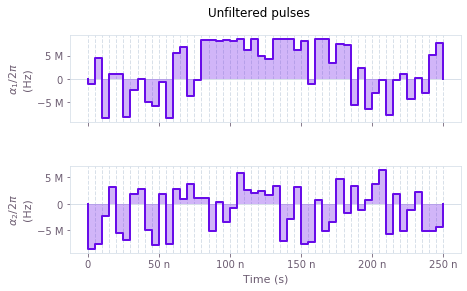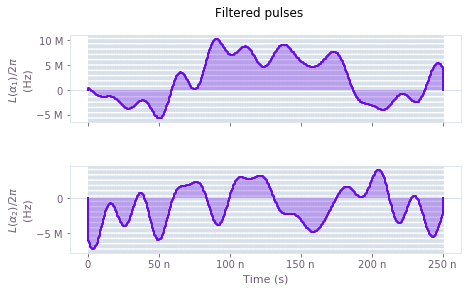## Example: CRAB optimization

In chopped random basis (CRAB) optimization, pulses are defined via optimizable linear combinations from a set of basis functions, which can greatly reduce the dimensionality of the optimization search space. Traditionally, a randomized Fourier basis is used, although the same technique has also seen success with other bases, for example Slepian functions. In this example, we perform a CRAB optimization (in the Fourier basis) of a qutrit system in which we effect a single-qubit gate while minimizing leakage out of the computational subspace. The system is described by the following Hamiltonian:

\begin{align*} H(t) = & \frac{\chi}{2} (a^\dagger)^2 a^2 + \gamma(t) (1+\beta(t))a + \gamma^*(t)(1+\beta(t)) a^\dagger + \frac{\alpha(t)}{2} a^\dagger a \,, \end{align*}

where $\chi$ is the anharmonicity, $\gamma(t)$ and $\alpha(t)$ are, respectively, complex and real time-dependent pulses, $\beta$ is a small, slowly-varying stochastic amplitude noise process, and $a = |0 \rangle \langle 1 | + \sqrt{2} |1 \rangle \langle 2 |$.

The Q-CTRL optimization engine provides a convenience function, qctrl.operations.real_fourier_pwc_signal, for creating optimizable signals in a Fourier basis, suitable for use in a CRAB optimization. Other bases are supported by the framework, but require the user to manually provide operations that compute the appropriate linear combinations.

# Define standard matrices
a = np.array([[0., 1., 0.],
[0., 0., np.sqrt(2)],
[0., 0., 0.]], dtype=np.complex)
[1., -1.,0],
[0, 0, np.sqrt(2)]], dtype=np.complex)/np.sqrt(2)
qubit_projector = np.array([[1, 0, 0],
[0, 1, 0],
[0, 0, 0]], dtype=np.complex)

# Define physical constraints
chi = 2 * np.pi * -300.0 * 1e6 #Hz
gamma_max = 2 * np.pi * 30e6 #Hz
alpha_max = 2 * np.pi * 30e6 #Hz
segment_count = 200
duration = 100e-9 #s

# Create graph object
with qctrl.create_graph() as graph:
# Create gamma(t) signal in Fourier bases. To demonstrate the full
# flexibility, we show how to use both randomized and optimizable
# basis elements. Elements with fixed frequencies may be chosen too.
gamma_i = qctrl.operations.real_fourier_pwc_signal(
duration=duration,
segments_count=segment_count,
randomized_frequencies_count=10,
)
gamma_q = qctrl.operations.real_fourier_pwc_signal(
duration=duration,
segments_count=segment_count,
optimizable_frequencies_count=10,
)
gamma = qctrl.operations.pwc_signal(
duration=duration,
values=qctrl.operations.complex_value(real=gamma_i.values, imag=gamma_q.values) * gamma_max,
name="gamma",
)

# Create alpha(t) signal
alpha = qctrl.operations.real_fourier_pwc_signal(
duration=duration,
segments_count=segment_count,
initial_coefficient_lower_bound=-alpha_max,
initial_coefficient_upper_bound=alpha_max,
optimizable_frequencies_count=10,
name="alpha",
)

# Create anharmonicity term
anharmonicity = qctrl.operations.constant_pwc_operator(
duration, ad2a2 * chi / 2,
)
# Create drive term
drive = qctrl.operations.pwc_operator_hermitian_part(
qctrl.operations.pwc_operator(signal=gamma,
operator=2*a)
)

# Create clock shift term
shift = qctrl.operations.pwc_operator(signal=alpha,

# Create infidelity (note that we project the target operator to the
# qubit subspace in order to calculate infidelity only in the subspace,
# and set a filter function projector in order to evaluate robustness
# only from the subspace).
target_operator = qctrl.operations.target(
filter_function_projector=qubit_projector
)

infidelity = qctrl.operations.infidelity_pwc(
hamiltonian=qctrl.operations.pwc_sum([anharmonicity, drive, shift]),
target_operator=target_operator,
noise_operators=[drive],
name="infidelity",
)

# Run the optimization
optimization_result = qctrl.functions.calculate_optimization(
cost_node_name="infidelity",
output_node_names=["alpha", "gamma"],
graph=graph,
)

print("Optimized cost:\t", optimization_result.cost)

# Plot the optimized controls
plot_controls(plt.figure(),
controls={"$\\alpha$": optimization_result.output["alpha"],
"$\\gamma$": optimization_result.output["gamma"]})
plt.show()

100%|██████████| 100/100 [01:59<00:00,  1.20s/it]

Optimized cost:	 1.2697311341110484e-08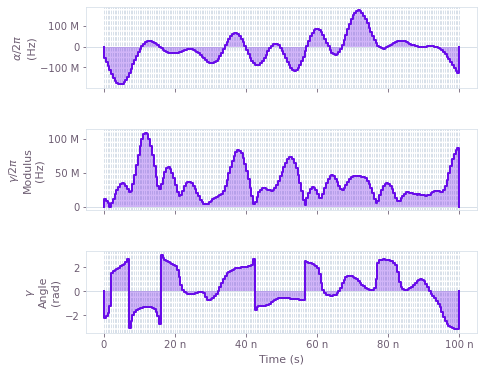## Example: estimating system parameters

Next we show how to use the optimizer to estimate unknown parameters in a system. A single-qubit system is subject to a Hamiltonian of the form

\begin{equation*} H = \Omega \sin(\theta) \sigma_{x} + \Omega \cos(\theta) \sigma_{z} , \end{equation*}

for a fixed time duration, and while the (constant) amplitude $\Omega$ can be controlled, the (constant) parameter $\theta$ is unknown (but fixed between realizations). The aim is to estimate the value of $\theta$ from some measurements $\{M_i\}$ (for instance, of the infidelity of a spin flip) that have been obtained after the system has evolved under a set of amplitude values, $\{\Omega_i\}$.

The optimizer can be used to estimate $\theta$ by trying to find the form of the evolution that would most closely match the measured points. For a given $\theta$, the expected measurements from the system $\{m_i\}$ can be compared to the actual measurements $\{M_i\}$ via the cost function

\begin{equation*} C = \sum_i \frac{[M_i - m_i(\theta)]^2}{2 (\Delta M_i)^2} , \end{equation*}

where $\Delta M_i$ is the standard deviation of the measured input point $M_i$. Assuming a Gaussian probability distribution for the errors in the measurements, minimizing this cost function is equivalent to minimizing the negative log likelihood that a value of $\theta$ could generate the points $\{M_i\}$, yielding the best choice of $\theta$ that reproduces the original measurements. You can also estimate the precision of the obtained parameter by using the Cramér–Rao bound to calculate the covariance of the estimated variable from the inverse of the Hessian of this cost function.

In order to implement the cost function you can create an optimizable variable $\theta$, and use it to build the system's Hamiltonian. You can then calculate the evolution of the system for each value of $\Omega$, the corresponding spin-flip infidelities, and their associated cost. Note that you can perform the calculation for all the infidelities in a single batch, resulting in a more efficient calculation compared with using a loop. See the qctrl.operations.pwc_signal function to learn more about batches in the Q-CTRL optimization engine. The engine also provides the convenience function qctrl.operations.hessian_matrix to calculate the Hessian of the cost, as well as mathematical functions to perform on the graph nodes, such as qctrl.operations.sin and qctrl.operations.cos.

See the Control hardware: system identification Application note for more examples on system identification.

omega_values = np.array(
[3.513e6, 3.353e6, 3.612e6, 3.555e6, 3.293e6, 2.949e6, 2.688e6, 2.876e6, 3.110e6]
)
measurements = np.array(
[0.902, 0.971, 0.845, 0.904, 0.982, 0.967, 0.879, 0.954, 0.979]
)
measurement_errors = np.array(
[0.018, 0.012, 0.019, 0.009, 0.020, 0.012, 0.017, 0.015, 0.010]
)

# Define standard matrices
sigma_x = np.array([[0, 1], [1, 0]], dtype=np.complex)
sigma_z = np.array([[1, 0], [0, -1]], dtype=np.complex)

# Define physical constant
duration = 1e-6  # s

# Define parameter to be estimated
actual_theta = 0.3 * np.pi

# Create graph object
with qctrl.create_graph() as graph:

# Create the parameter we want to estimate
theta = qctrl.operations.bounded_optimization_variable(
count=1, lower_bound=0.0, upper_bound=np.pi / 2.0, name="theta"
)

# Create (batched) signals for the sigma_x and sigma_z terms
# The last dimension of the pwc signals corresponds to time, and any
# preceding dimensions to the batches (here, a single one for the omegas).
# The batching is preserved through the computation.
alpha_x = qctrl.operations.pwc_signal(
values=qctrl.operations.sin(theta) * omega_values[:, None],
duration=duration,
)
alpha_z = qctrl.operations.pwc_signal(
values=qctrl.operations.cos(theta) * omega_values[:, None],
duration=duration,
)

# Create Hamiltonian by adding both terms
hamiltonian = qctrl.operations.pwc_sum(
terms=[
qctrl.operations.pwc_operator(signal=alpha_x, operator=sigma_x),
qctrl.operations.pwc_operator(signal=alpha_z, operator=sigma_z),
]
)

# Calculate the spin-flip infidelities
populations = qctrl.operations.infidelity_pwc(
hamiltonian=hamiltonian,
target_operator=qctrl.operations.target(operator=sigma_x),
)

# Calculate the cost
cost = qctrl.operations.sum(
(populations - measurements) ** 2.0 / (2.0 * measurement_errors ** 2.0),
name="cost",
)

# Calculate the Hessian matrix
hessian = qctrl.operations.hessian_matrix(cost, [theta], name="hessian")

# Run the optimization
optimization_result = qctrl.functions.calculate_optimization(
cost_node_name="cost",
output_node_names=["theta", "hessian"],
optimization_count=10,
graph=graph,
)

# Retrieve the results of the optimization
print("Optimized cost:\t", optimization_result.cost)
calculated_theta = optimization_result.output["theta"]["value"]
hessian = optimization_result.output["hessian"]["value"]

# Calculate the uncertainty from the inverse of the Hessian matrix
uncertainty_theta = np.sqrt(np.linalg.inv(hessian))[0,0]

print(
f"Obtained theta:\t ({calculated_theta/np.pi:.4f} ± {uncertainty_theta/np.pi:.4f})π"
)
print(f"Actual theta:\t {actual_theta/np.pi}π")

100%|██████████| 100/100 [00:08<00:00, 11.54it/s]
Optimized cost:	 3.769966575632828
Obtained theta:	 (0.2986 ± 0.0129)π
Actual theta:	 0.3π